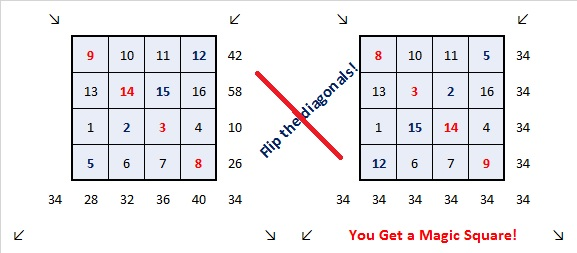# 880 Flip the Diagonals!

There are 880 different 4 × 4 Magic Squares. (In addition, each of those can be rotated and/or reflected to make 880 × 8 Magic Squares.) I have found a few that start with some simple ways to order the numbers 1 to 16 in the boxes and then flip the diagonals to create a perfect Magic Square.

For this first one I began by putting the numbers 1-4 in one box with the numbers 13-16 in the cater-corner box. Then 5-8 and 9-12 occupy the other spaces. Then I flipped the diagonals and got a Magic Square!If we flip the locations of the numbers 5-8 and the numbers 9-12, it still works:This third Magic Square begins with the most common way to order the numbers from 1 to 16:With just a little tweaking of that most common way to order the numbers, I was able to make the following three Magic Squares. Begin by switching the first two rows with each other and the last two rows with each other. Notice in every beginning square the diagonals already equal 34.We can also switch the first two rows with the last two rows, but that just gives us a reflection of the one just above it. (The colors don’t match, but it is still a reflection!)Try switching the first two columns with the last two columns. You’ll get another different one.There are MANY more ways to do a 4 × 4 Magic Square.

Last week’s excel file, 12 factors 864-874, included some Magic Square templates including the 4 × 4 one. You can use that file to find more Magic Squares. Go ahead give it a try!

Here’s a little more about the number 880:

880 is the hypotenuse of a Pythagorean triple:

• 528-704-880, which is (3-4-5) times 176.

880 is the sum of the twelve prime numbers from 47 to 101.

• 880 is a composite number.
• Prime factorization: 880 = 2 × 2 × 2 × 2 × 5 × 11, which can be written 880 = 2⁴ × 5 × 11
• The exponents in the prime factorization are 4, 1 and 1. Adding one to each and multiplying we get (4 + 1)(1 + 1)(1 + 1) = 5 × 2 × 2 = 20. Therefore 880 has exactly 20 factors.
• Factors of 880: 1, 2, 4, 5, 8, 10, 11, 16, 20, 22, 40, 44, 55, 80, 88, 110, 176, 220, 440, 880
• Factor pairs: 880 = 1 × 880, 2 × 440, 4 × 220, 5 × 176, 8 × 110, 10 × 88, 11 × 80, 16 × 55, 20 × 44 or 22 × 40
• Taking the factor pair with the largest square number factor, we get √880 = (√16)(√55) = 4√55 ≈ 29.66479.This site uses Akismet to reduce spam. Learn how your comment data is processed.